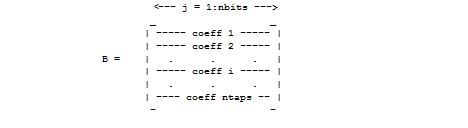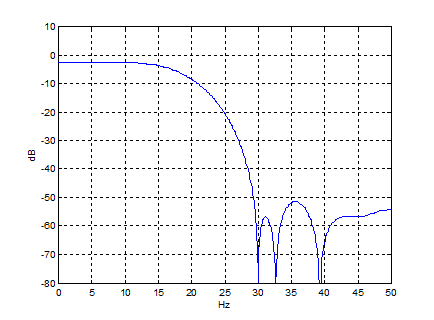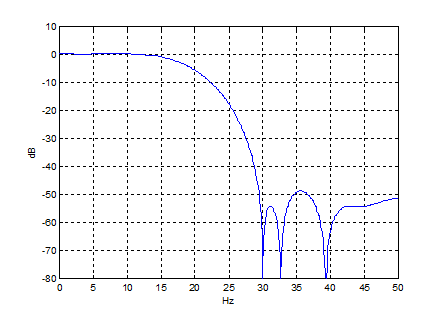# Matlab Code to Synthesize Multiplierless FIR Filters

This article presents Matlab code to synthesize multiplierless Finite Impulse Response (FIR) lowpass filters.

A filter coefficient can be represented as a sum of powers of 2.  For example, if a coefficient = decimal 5 multiplies input x, the output is $y= 2^2*x + 2^0*x$.  The factor of $2^2$ is then implemented with a shift of 2 bits.  This method is not efficient for coefficients having a lot of 1’s, e.g. decimal 31 = 11111.  To reduce the number of non-zero bits, we introduce a signed-digit approach, and allow each bit to be -1, 0, or +1.  Then the value 31 becomes:

$31 = 32 – 1 = 2^5 - 2^0$

Each coefficient is realized as shifts and signed sums.

There are several possible ways to generate signed digits.  The Canonic Signed-Digit (CSD) representation is typically used.  The CSD value is unique, and has the feature that no adjacent digits are both nonzero.  That is, the combinations 11, -11, 1-1, or -11 do not occur.

We can write 31 as a 6-digit CSD value:

31 = 1 0 0 0 0 -1

Thus a binary number with five 1’s can be represented as CSD with two nonzero digits.   The approach used to synthesize CSD lowpass filters is described below.

## CSD Filter Synthesis

I chose the approach used here because it is simple and reliable.  Other methods may yield fewer nonzero digits, but require more complex optimization methods.  The two m-files used for filter synthesis are listed in Appendix A.  The general steps to synthesize the CSD coefficients are as follows:

• Synthesize low-pass filter floating-point coefficients using the Parks-McClellan algorithm.  This step uses the Matlab command firpm(N,f,a)   (formerly remez(N,f,a)).
• Choose a scaling -- i.e. maintap value -- for the coefficients.  Round the scaled floating-point coefficients to integers using the desired number of bits.
• Convert the integer coefficients to CSD – this is an exact conversion, so the CSD filter has exactly the same response as the integer filter.  The conversion algorithm is described in .

Scaling the coefficients affects the number of signed digits in an unpredictable way.  We want to minimize the number of signed digits in the coefficients.  To do this, the m-file searches over several maintap values and computes CSD coefficients for each case, then finds a minimum based on the sum of signed digits in the coefficients (see the Matlab function description below for more detail).

Note that for a coefficient length of nbits, the largest possible CSD value is 1010 … .  As an example, for an 8-bit coefficient, this value is 27 + 25 + 23 + 21 = 170.  Defining full-scale as 28, the largest CSD value is:

CSD_max = 170/28  = 0.66406 of full-scale.    (8 bit coeff.)

For large nbits, CSD_max approaches 2/3 of full-scale.   Thus, when selecting maintap values, we must stay below 2/3 *2nbits.

The method described above is not limited to conventional FIR lowpass filters.  For example, we could also synthesize halfband, bandpass, or highpass filters using this approach.

## Matlab function csd_lowpass

Purpose

Design of Canonic-signed-digit (CSD) FIR low-pass filters.

Syntax

    [b_opt,B]= csd_lowpass(ntaps,nbits,fpass,fstop,fs);
ntaps     number of FIR coefficients
nbits     number of bits per CSD coefficient
fpass     passband edge
fstop     stopband edge
fs        sample frequency
b_opt    vector of coefficients in decimal integer format
B        matrix of CSD coefficients in binary format, MSB on right.Description

csd_lowpass synthesizes an FIR lowpass filter with CSD coefficients.  It uses the Parks-McClellan filter design function firpm to design a floating-point lowpass filter.  The floating-point coefficients are quantized to nbits bits and then converted to CSD.

The coefficients of the filter are scaled to minimize the number of signed digits (nsd) according to the following criteria:

• If nbits < 11, coefficients are scaled to minimize those with nsd greater than 2.
• If nbits >=11, coefficients are scaled to minimize those with nsd greater than 3.

The output b_opt is a vector of coefficients in decimal integer format.  The output B is a matrix containing the equivalent CSD coefficients in binary signed-digit format.  Each row of B contains a coefficient of length nbits with the MSB on the right.

Assuming a hardware implementation with nbits+1 right-shifts, the dc gain of the filter is given by:

dc gain = sum(b_opt)/2nbits+1

Thus, to achieve overall unity dc gain, an external gain stage with gain_ext = 2nbits+1/sum(b_opt) can be used.  gain_ext is displayed in the workspace.

Note:  The results of the coefficient search are sensitive to the input parameters fpass and fstop.  It may be possible to reduce some coefficients’ number of signed digits by making small changes to fpass or fstop.

Subroutine

csd_lowpass calls the function dec2csd1 to compute the CSD values (see Appendix A).

Example

Design a CSD LPF with 17 9-bit coefficients that has 10 Hz passband, 30 Hz stopband, and fs = 100 Hz.

    ntaps= 17;
nbits= 9;
fpass= 10;
fstop= 30;
fs= 100;
b_opt= csd_lowpass(ntaps,nbits,fpass,fstop,fs);


The output to the workspace is shown below.  coeff denominator is equal to2nbits+1.   Exact and rounded (approximate) external gain values are provided.  The approximate value can be used for multiplierless implementation of the gain.

The coefficients are displayed in decimal integer and binary CSD formats.  The number of signed digits (nsd) in each CSD coefficient is listed to its right.  The CSD coefficient bits are displayed with MSB on the left (this is opposite to the order in the matrix B).

    coeff denominator = 1024
external gain for unity overall gain: 1.3581
approximate external gain =     11/8
fixed-point coeff values
-1
2
10
1
-33
-36
60
222
304
222
60
-36
-33
1
10
2
-1
CSD coeffs, MSB on left;    nsd
0  0  0  0  0  0  0  0 -1     1
0  0  0  0  0  0  0  1  0     1
0  0  0  0  0  1  0  1  0     2
0  0  0  0  0  0  0  0  1     1
0  0  0 -1  0  0  0  0 -1     2
0  0  0 -1  0  0 -1  0  0     2
0  0  1  0  0  0 -1  0  0     2
1  0  0 -1  0  0  0 -1  0     3
1  0  1  0 -1  0  0  0  0     3
1  0  0 -1  0  0  0 -1  0     3
0  0  1  0  0  0 -1  0  0     2
0  0  0 -1  0  0 -1  0  0     2
0  0  0 -1  0  0  0  0 -1     2
0  0  0  0  0  0  0  0  1     1
0  0  0  0  0  1  0  1  0     2
0  0  0  0  0  0  0  1  0     1
0  0  0  0  0  0  0  0 -1     1


Plot the filter response without the external gain stage:

    [h,f]= freqz(b_opt/1024,1,1024,fs);
H= 20*log10(abs(h));
plot(f,H),grid, axis([0 fs/2 -80 10])
xlabel('Hz'),ylabel('dB')Figure 1.  Response without external gain stage

Plot the filter response with the approximate external gain value of 11/8:

    bg= b_opt/1024 * 11/8;
[h,f]= freqz(bg,1,1024,fs);
H= 20*log10(abs(h));
plot(f,H),grid, axis([0 fs/2 -80 10])
xlabel('Hz'),ylabel('dB')Figure 2.  Response with approximate external gain value = 11/8

## References

1.  “Parks-McClellan optimal FIR filter design”, Mathworks website.

2.  Ruiz and Granda, "Efficient Canonic Signed Digit Recoding",  Microelectronics Journal 42, p 1090-1097, 2011

## Appendix A.  Matlab Functions for CSD lowpass FIR filter synthesis

These programs are provided as-is without any guarantees or warranty.  The author is not responsible for any damage or losses of any kind caused by the use or misuse of the programs.

There are two Matlab functions, csd_lowpass and dec2csd1.  csd_lowpass calls dec2csd1.

1.  csd_lowpass

%function [b_opt,B]= csd_lowpass(ntaps,nbits,fpass,fstop,fs)
% 10/30/2016 Neil Robertson
%
% Synthesize FIR LPF with CSD coeffs
%
% ntaps     number of FIR coeffs
% nbits     number of bits per coeff
% fpass     passband edge freq, Hz, kHz, or MHz
% fstop     stopband edge freq, Hz, kHz, or MHz
% fs        sample freq, Hz, kHz, or MHz
%
% b_opt     decimal integer coefficients of LPF
% B         CSD coefficients of LPF (exactly equivalent to b_opt)
%
% Examples
% csd_lowpass(17,9,10,30,100);
% csd_lowpass(27,11,10,25,100);
%
function [b_opt,B]= csd_lowpass(ntaps,nbits,fpass,fstop,fs)
% 1.  Synthesize floating-point LPF using Parks-McClellan algorithm
N= ntaps-1;
f= [0 fpass fstop fs/2]/(fs/2);  % frequency vector
a= [1 1 0 0];                    % response goal vector
b= firpm(N,f,a);                 % Parks-McClellan filter synthesis
%b= remez(N,f,a);
% 2.  SEARCH for CSD coeffs with lowest number of signed digits (nsd)
b= b/max(b);                  % make maintap= 1
nsd_thresh= 2;                % threshold used to compute error
if nbits > 10
nsd_thresh=3;
end
stop= fix(2/3*2^nbits);       % max allowed CSD value for coeff of length nbit
start= max(2^(nbits-1),stop-600); % starting maintap value. start > stop -600
emin= 999999;
for maintap= start:stop
b_int=round(b*maintap);      % decimal integer coefficients
Y= dec2csd1(b_int,nbits);     % compute CSD representation of b_int
for i=1:ntaps
nsd(i)= sum(abs(Y(i,:)));     % number of signed digits in coeff i
end
m= find(nsd> nsd_thresh);     %find indeces of coeffs that have nsd > nsd_thresh
e= sum(nsd(m));               % sum of nsd's for those coeffs
if e <=emin
emin= e;
Yopt= Y;                   % CSD coeffs with least nsd's.
b_opt= b_int;              % integer version of above
end
end
%
% 3.  Compute nsd of coeffs and external gain value
for i= 1:ntaps
nsd(i)= sum(abs(Yopt(i,:)));        % number of signed digits in coeff i
end
gain_ext= 2^(nbits+1)/sum(b_opt);    % gain to make overall dc gain = 1
gain_approx= round(gain_ext*16)/16;    % approx gain
gain_rat= rats(gain_approx);
disp(' ')
disp(['coeff denominator = ',num2str(2^(nbits+1))])
disp(['external gain for unity overall gain: ',num2str(gain_ext)])
disp(['approximate external gain =',num2str(gain_rat)])
% 4.  List coeff values in decimal and CSD formats
disp(' ')
disp('fixed-point coeff values')
disp(b_opt')
B = [fliplr(Yopt)];
disp('CSD coeffs, MSB on left;    nsd')
disp(' ')
for i= 1:ntaps
disp([num2str(B(i,:)),'     ',num2str(nsd(i))])
end



2.  dec2csd1

% Y= dec2csd1(b_int,nbits)          10/25/16  Neil Robertson
%
% Convert signed decimal integers to binary CSD representation
% See Ruiz and Granda, "Efficient Canonic Signed DigitRecoding",
%      Microelectronics Journal 42, p 1090-1097, 2011
%
% b_int = vector of decimal integer coefficients
% nbits = number of bits in b_int coeffs
% Y = matrix of CSD coeffs
% A = matrix of binary coeffs
%
%                    -- j= 1:nbits --
%                   _                 _
%                  | ---- coeff 1 ---- |
%                  | ---- coeff 2 ---- |
%                  |  .      .      .  |
%       Y,A =      | ---- coeff i ---- |
%                  |  .      .      .  |
%                  | -- coeff ntaps -- |
%                   -                 -
% 1.  convert decimal integers to binary integers
function Y= dec2csd1(b_int,nbits)
ntaps= length(b_int);       % number of coeffs
for i= 1:ntaps              % coeff index (row index)
u= abs(b_int(i));
for j= 1:nbits           % binary digit index (column index)
A(i,j)= mod(u,2);     % coeff magnitudes note:  MSB is on right.
u= fix(u/2);
end
end
% 2.  convert binary integers to CSD
s= sign(b_int);            % signs of coeffs
z= zeros(ntaps,1);
x= [A z];                  % MSB is on right. append 0 as MSB
for i= 1:ntaps                % coeff index (row index)
c= 0;
for j= 1:nbits         % binary digit index (column index)
d= xor(x(i,j),c);
ys= x(i,j+1)&d;               % sign bit    0 == pos, 1 == negative
yd= ~x(i,j+1)&d;              % data bit
Y(i,j)= yd - ys;              % signed digit
c_next = (x(i,j)&x(i,j+1)) | ((x(i,j)|x(i,j+1))&c);      % carry
c= c_next;
end
Y(i,:) = Y(i,:)*s(i);            % multiply CSD coeff magnitude by coeff sign
end


## Appendix B.  Demonstration of Binary to CSD conversion algorithm

%bin2csd_demo.m          nr 10/26/16
% Convert an unsigned binary number to CSD
% See Ruiz and Granda, "Efficient Canonic Signed Digit Recoding"
%     Microelectronics Journal 42, p 1090-1097, 2011
%
% logical operators:
%     &  AND
%     |  OR
%     ~  NOT
% Definition of ys and yd:
%
%  y    ys  yd
%  0    0    0
%  1    0    1
% -1    1    0
%
% So given ys and yd: y = yd - ys
% positive binary number, MSB on left
bin= [0 1 1 1];
%bin= [0 0 1 0 1 0 1 1 1];       % example from Ruiz paper
N= length(bin);
x= [fliplr(bin) 0];        % MSB is on right, i.e. x(1)= LSB, x(N+1)= MSB = 0
c= 0;
for j= 1:N
d= xor(x(j),c);
ys= x(j+1)&d;     % sign bit
yd= x(j+1)&d;    % data bit
y(j)= yd- ys;    % signed digit
c_next = (x(j)&x(j+1)) | ((x(j)|x(j+1))&c);     % carry
c= c_next;
end
fliplr(y)                     % display MSB on left
% check csd value
i= 0:N-1;
a= 2 .^i;
csd_check= sum(a.*y)


[ - ]
Comment by November 5, 2016Thanks for the article. In fpgas though we have so many multipliers and rarely resort to seek alternatives.  In some modern fpgas there are multiply-add blocks that in effect end up with fir filter implemented almost as an asic with little use of fabric logic achieving very high speed.

However, in very few cases I have seen some designs using additions + preadd of symmetry and end up with good design but this uses plenty of fabric and this could be ok if we want to balance the available resource. Your approach will certainly help in such cases.

The other side effect is that we don't want to lose bit resolution or end up with rigid structure that is not easily configurable. we also need to correct for gain.

Thanks

Kaz

[ - ]
Comment by November 5, 2016Kaz,

You're welcome.  I must admit I'm not conversant with FPGA's, coming from an RF background.

regards,

Neil

P.S.  I should mention that these CSD filters are useful at high sample frequencies -- the last design in which I was involved had CSD filters with sample rates above 200 MHz.

[ - ]
Comment by August 16, 2018Neil , Thanks for the article. Great stuff.  Some FPGA do not have multiplier resources or enough of them. I've been working with FGPA for  25 years now.

To post reply to a comment, click on the 'reply' button attached to each comment. To post a new comment (not a reply to a comment) check out the 'Write a Comment' tab at the top of the comments.